# Texas Go Math Grade 1 Lesson 14.4 Answer Key Compose More Shapes

Refer to our Texas Go Math Grade 1 Answer Key Pdf to score good marks in the exams. Test yourself by practicing the problems from Texas Go Math Grade 1 Lesson 14.4 Answer Key Compose More Shapes.

## Texas Go Math Grade 1 Lesson 14.4 Answer Key Compose More Shapes

Explore

Use shapes to fill each outline. Draw to show your work.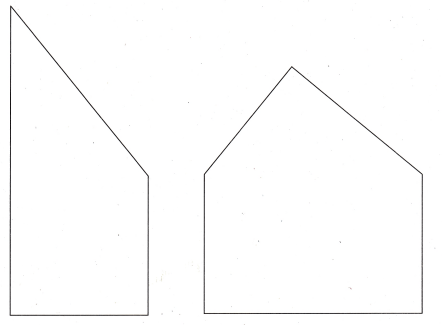Answer: The work has shown in the below diagram.
Explanation:After drawing the outline then the first diagram will become a right angle triangle, square. And the second diagram becomes a triangle and a rectangle.

FOR THE TEACHER • Have children use two shapes to fill the outline on the left, and draw a line to show the two shapes. Then have children use three shapes to fill the outline on the right, again drawing lines to show the shapes.
Answer: Children can make squares and rectangles because those two shapes have a chance to fill the outline on the left side. This work can be shown in the below diagram.
Explanation:The left outline had drawn in the figure. And another thing in the question asked was the need to draw 3 shapes to fill the outline right side.They can make many shapes like a parallelogram, trapezium etc.

Math Talk
Mathematical Processes

Use the outline on the left to describe how two shapes can make another shape.
Answer: We can make a rectangle by using two squares.
Explanation:
By drawing the outline on the left we can make a rectangle. let’s see in the below diagram.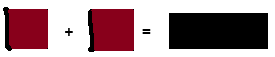Model and Draw

Combine shapes to make a new shape.How else could you combine 2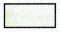?
Answer: We can make 4 squares and one rectangle, one square, 3 triangles.
Explanation:
It’s according to our division of a rectangle. So I divided the rectangle into 4 parts then it becomes 4 squares. And in other rectangles are also divided with possible outlines. The diagram is shown below.Share and Show

Circle two shapes that can combine to make the shape on the left.

Question 1.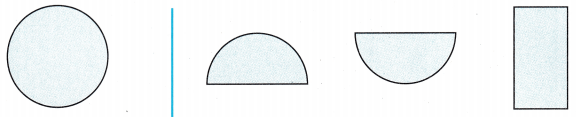Answer: The two semicircles are needed to complete the whole shape which is called the circle.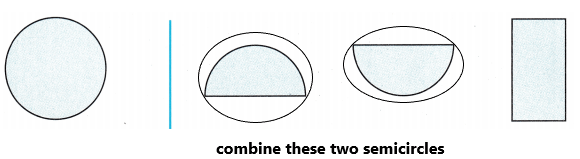Question 2.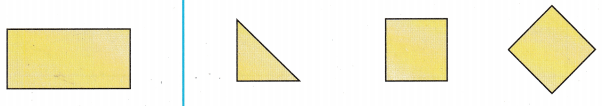Answer: The two squares are needed to complete the whole shape which is called the rectangle.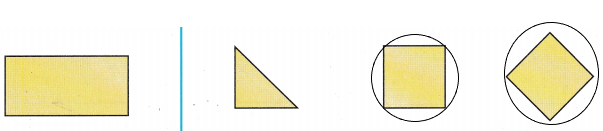Question 3.Answer: The two arcs are needed to complete the whole shape.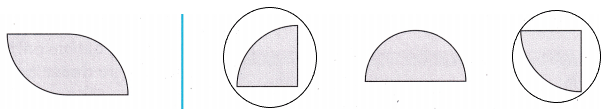Problem Solving

Circle two shapes that can combine to make the shape on the left.

Question 4.Answer: One arc and one square are needed to complete the whole shape.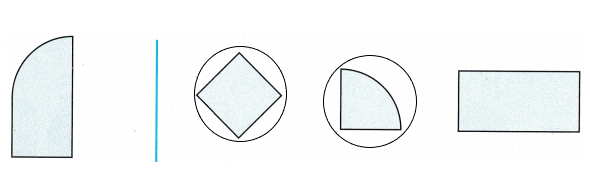Question 5.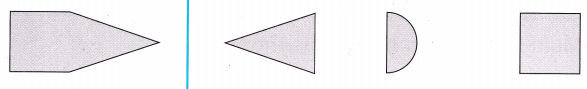Answer: One square and one triangle are needed to complete the whole shape.H.O.T. Multi-Step Draw lines to show two different ways to combine the shapes on the left to make new shapes on the right.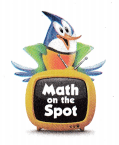Question 6.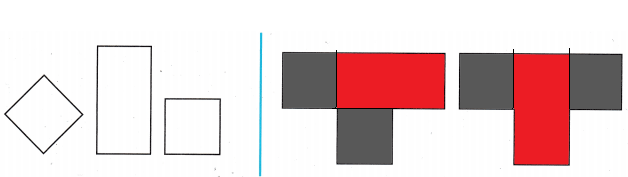Question 7.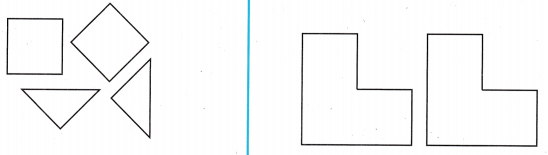We need to think about how to put these shapes in the given block and then make different shapes.

Question 8.
Analyze Which shapes can combine to make a circle?Answer: The first option is correct.Question 9.
Multi-Step Alana builds this shape. Esther takes the shape apart. She uses the same blocks. Which new shape could Esther build?Answer: The last option is correct.
Explanation: In the given shape we are having 6 triangles and two hexagons.Question 10.
Texas Test Prep Which shapes can combine to make this new shape?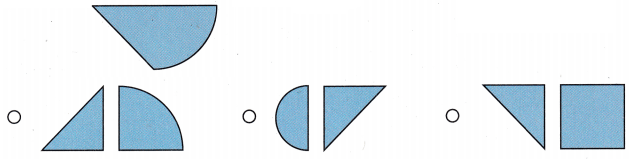Answer: The middle one is correct.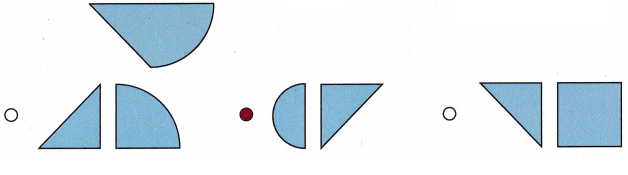TAKE HOME ACTIVITY • Ask your child to draw a new shape he or she can make by combining two triangles.
Answer: A square can be subdivided into two congruent isosceles right triangles.
Two congruent isosceles right triangles can be combined to form a square.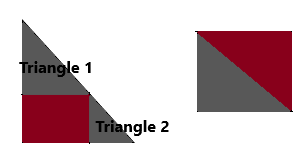### Texas Go Math Grade 1 Lesson 14.4 Homework and Practice Answer Key

Circle two shapes that can combine to make the shape on the left.

Question 1.Answer: The representation has been done in the below diagram.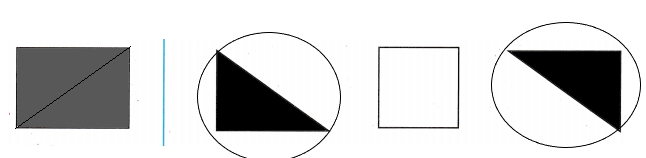Question 2.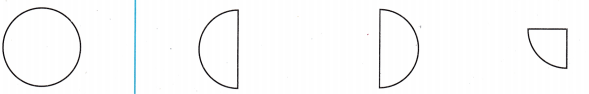Answer: The representation has been done in the below diagram.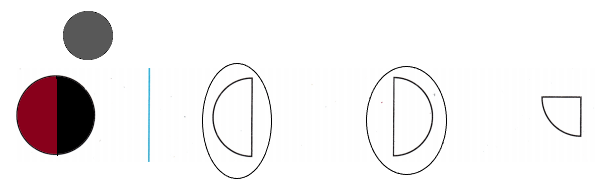Problem Solving

Multi-Step Draw lines to show two different ways to combine the shapes on the left to make new shapes on the right.

Question 3.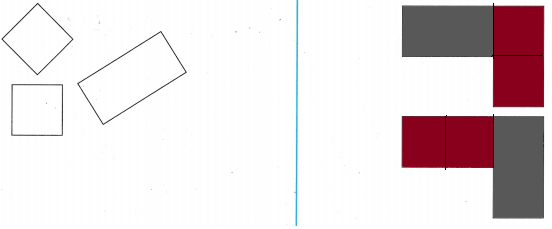We need to think about how to put these shapes in the given block and then make different shapes.

Lesson Check

Question 4.
Which shapes can combine to make a square?Answer: The first one is correct.Divide the square into two parts then we will get two triangles. So, If we join two triangles here we will get a square.

Question 5.
Which shapes can combine to make this new shape?Answer: The last one is correct.
Explanation:
The division of shape is shown in the below diagram.Scroll to Top